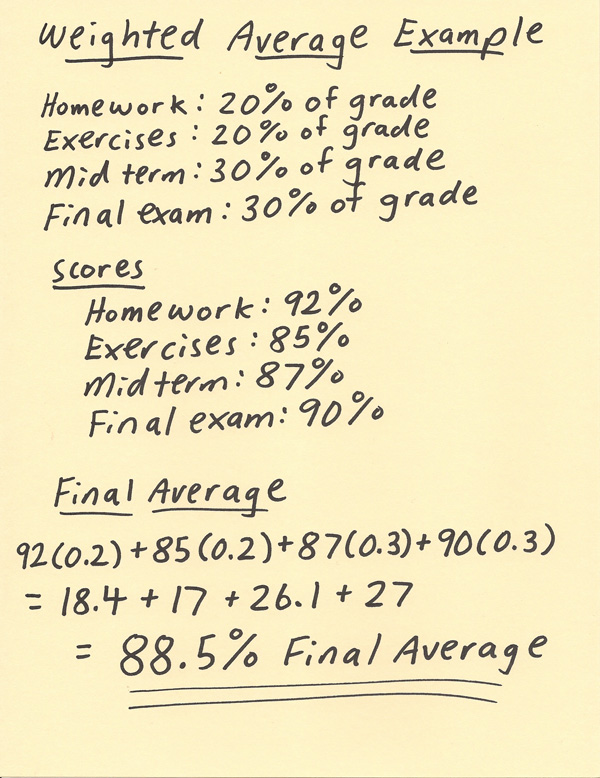[--MAIN HOME--] [--ALL HABYHINTS--] [--FACEBOOK PAGE--]

 CALCULATING WEIGHTED AVERAGE

METEOROLOGIST JEFF HABY

A straight forward average is adding the numbers and dividing by the total number of numbers. For example, the average of the numbers 4.0, 7.0, 11.0, 3.0 and 6.0 is (4.0 + 7.0 + 11.0 + 3.0 + 6.0) / 5 = 6.2

Another term for the average is the mean.

In a weight average situation, each portion of the grade will have a different percentage of the total grade or weight to the grade. For example, suppose tests are 75% of the grade and homework is 25% of the grade. If the test average is 88% and the homework average is 96%, then the weight average is 88*(0.75) + 96*(0.25) = 66 + 24 = 90%.

When taking a class or when working with weight average data, it is important to do the calculation correctly because if it is done incorrectly then this will result in the incorrect grade or incorrect statistic. Here are some pointers about weighted averages:

1) If the numbers are weighted differently, then a straight forward average of taking all the numbers, adding them and dividing by the total number of numbers will not work. The weight of each score will need to be determined first before calculating the final grade.

2) The portions of the grade that have the highest percent weight will impact the grade the most. Thus, it is more important to score a higher grade on the assignments that are worth a higher percentage of the grade. However, each portion of the grade will have an impact on the final grade. Thus, try for the highest score on each portion of the grade.

3) Real life applications where weight averages are used include the calculation of a credit score, calculation of GPA, final grade calculations, some sports statistics, college entrance requirements, certain judging methods such as in sports and job performance, job hiring qualifications and exams where questions are not worth the same number of points.

Below is an example of a weighted average to find the final grade in a course based on how much each portion of the total grade is worth and the average or score of each component of the grade. Note that some of the scores likely required a simple average to get that component. For example, the homework average of 92% below likely would result from the average of several homework scores over the course of the semester.